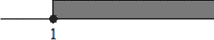# Improper Fraction

Let you have a fraction a ⁄ b, then how can you know if it's an improper fraction?

It's easy! Look at "a", then look at "b". What is greater?

If "a" is greater than "b" (a > b), or "a" equals "b" (a = b), it means this time you have an improper fraction.

##### Example:

4 ⁄ 3 (4 > 3)     10 ⁄ 7 (10 > 7)     15 ⁄ 15 (15 = 15)

### It's interesting!

An improper fraction as a number has its own place on the number scale.

Every positive improper fraction is always greater than "1", or it equals "1".
(7 ⁄ 3 > 1; 7 ⁄ 7 = 1)But every negative improper fraction is always less than "-1", or it equals "-1".
(-7 ⁄ 3 < -1; -7 ⁄ 7 = -1)

### And what about "0", "1", "-1"?

You can write these numbers by the following way:

0 = 0 ⁄ 12 – proper fraction (0 < 12)

1 = 13 ⁄ 13 – improper fraction (13 = 13)

-1 = 8 ⁄ -8 – improper fraction (8 > -8)

Ok, now some words about addition, subtraction, multiplication, division, raising to a power.

### How can you do it with improper fractions?

There are special rules for improper fractions, but you have to do multiplication, division, raising to a power with improper fractions the same way as with proper fractions.

### Multiplication

a ⁄ b × c ⁄ d = a × c ⁄ b × d ; b ≠ 0, d ≠ 0

##### Example:

9 ⁄ 5 × 7 ⁄ 2 = 9 × 7 ⁄ 5 × 2 = 63 ⁄ 10, (63 > 10) – improper fraction.

##### Notice!

Doing multiplication with improper fractions you always get an improper fraction.

### Division

a ⁄ b ÷ c ⁄ d = a ⁄ b × d ⁄ c = a × d ⁄ b × c, c ≠ 0, d ≠ 0, b ≠ 0

##### Example:

5 ⁄ 3 ÷ 10 ⁄ 7 = 5 ⁄ 3 × 7 ⁄ 10 = 5 × 7 ⁄ 3 × 10 = 35 ⁄ 30, (35 > 30) - improper fraction.

17 ⁄ 17 ÷ 10 ⁄ 1 = 17 ⁄ 17 × 1 ⁄ 10 = 17 × 1 ⁄ 17×10 = 17 ⁄ 170 , (17 < 170) - proper fraction.

##### Notice!

When you divide improper fractions, you may get proper fraction or improper fraction.

### Rasing to a power

(a ⁄ b)n = an ⁄ bn, b ≠ 0

##### Example:

(5 ⁄ 2)2 = 52 ⁄ 22 = 25 ⁄ 4, (25 > 4) - improper fraction.

(10 ⁄ 3)4 = 104 ⁄ 34 = 10000 ⁄ 81, (10000 > 81) - improper fraction.

(7 ⁄ 7)10 = 710 ⁄ 710, (710 = 710) - improper fraction.

##### Notice!

When you do raising to a power with improper fraction, you always get only improper fraction.

You may do addition and subtraction with improper fractions like with proper ones. But, you'd better do it first making improper fractions as mixed numbers.

##### Example:

7 ⁄ 2 + 5 ⁄ 3 = (7 × 3 + 5 × 2) ⁄ 2 × 3 = (21 + 10) ⁄ 6 = 31 ⁄ 6

OR

7 ⁄ 2 + 5 ⁄ 3 = 3 + 1 ⁄ 2 + 1 + 2 ⁄ 3 = 3 + 1 + 1 ⁄ 2 + 2 ⁄ 3 = 4 + 7 ⁄ 6 = 4 + 1 + 1 ⁄ 6 =
5 + 1 ⁄ 6

##### Notice!

Every time you may do a mixed number from improper fraction and never the same with proper fraction!

Hope, now you see - "It is never late to learn".

#### Note

- fraction slash

= - is equal to; equals

- is not equal to; does not equal

< - is less than

> - is greater than

× - multiplied by

÷ - divided by